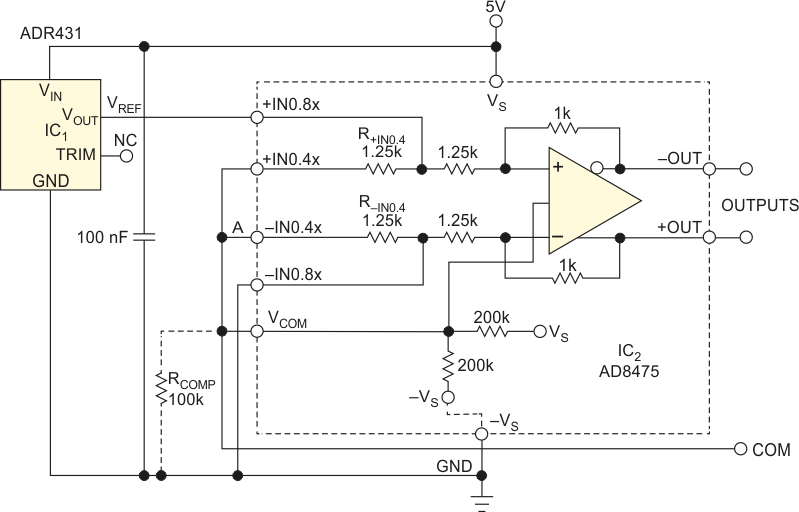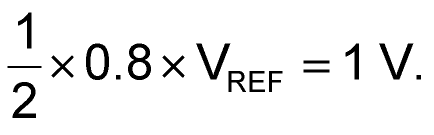# Build an accurate bipolar voltage reference

Marián Štofka

EDN

Pulse generators often need voltage comparators with accurately defined hysteresis, and such comparators need bipolar voltage references. Most voltage-reference-cell ICs are referenced to their low-side supply rail. If your circuit needs both positive and negative voltages, you could create the negative reference voltage by connecting a –1 gain inverter to the output of an IC reference cell. If your analog circuit runs from a single power supply, however, you must shift the common-mode voltage to a specific level. You can use the circuit in Figure 1 for that task.Figure 1. You can use this circuit to generate precision reference voltages of 1, –1, 1.25, and –1.25 V.

Reference cell IC1’s output voltage, VREF, connects to the noninverting input of amplifier IC2, an AD8475. This high-precision, differential-output, ×0.4/×0.8 amplifier in this case connects as an ×0.8 amplifier. The negative input, –IN, of IC2 is grounded. Voltages at its positive and negative outputs form the positive- and negative-output reference voltage, referenced to common-mode voltage VCOM. The magnitude of the generated reference voltages isThe AD8475’s gains of 0.4 and 0.8 have a tolerance of no more than 0.05% because of the device’s laser-trimmed internal gain-setting resistors. This circuit takes advantage of the AD8475’s gain-setting options. Typical use of the device as a ×0.8 amplifier keeps the +VIN0.4× and –VIN0.4× unconnected. In the circuit in Figure 1, however, these inputs interconnect, forming a high-precision 1-to-1 voltage divider of VREF.

The VCOM input of the AD8475 connects to this node, and the common-mode voltage of the generated reference voltages becomes VREF/2. Case A in Table 1 shows that the measured common-mode voltage is approximately 0.6% lower than VREF/2. This difference stems from the fact that the VCOM input connects within the AD8475 through one 200-kΩ resistor to the VS pin and through another 200-kΩ resistor to ground. You can, therefore, model the VCOM input as a source of VS/2 = 2.5 V with a series resistance of 100 kΩ. This series resistance acts as if it connects in parallel to the R+VIN0.4× resistor, which results in a slight imbalance in the 1-to-1 divider ratio. In cases B and C, the VCOM pin connects to Point A. The compensation resistor connects only in Case C. You can prove from the VGND values in cases B and C that RCOMP pushes the relative error of voltage at the COM output from 0.632% to –0.032%.

Table 1. Output voltages and voltage at VCOM pin
 Case A Case B Case C Positive-output voltage (V) 2.2525 0.9981 0.9986 Negative-output voltage (V) 0.2525 –1.0019 –1.0011 Ground voltage (V) –1.25 –1.2579 –1.2496 Common-mode voltage at VCOM pin (V) 1.239 0 0

In many applications, the magnitude of difference of bipolar reference voltages is important, and this imbalance has no effect on it. If, however, your application requires a high-accuracy common-mode voltage, you can connect a 100-kΩ compensation resistor between the VCOM pin and ground, and the circuit thus operates as in Case C. This approach almost fully retains the accuracy of the 1-to-1 divider ratio.

EDN